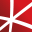# Top 20 NuGet statistics Packages

Foundational classes for financial, engineering, and scientific applications, including complex number classes, general vector and matrix classes, structured sparse matrix classes and factorizations, general sparse matrix classes and factorizations, general matrix decompositions, least squares solut...
Deedle implements an efficient and robust frame and series data structures for manipulating with structured data. It supports handling of missing values, aggregations, grouping, joining, statistical functions and more. For frames and series with ordered indices (such as time series), automatic align...
Math.NET Numerics is the numerical foundation of the Math.NET project, aiming to provide methods and algorithms for numerical computations in science, engineering and every day use. Supports .Net Framework 4.0 or higher and .Net Standard 1.3 or higher, on Windows, Linux and Mac.
F# Modules for Math.NET Numerics, the numerical foundation of the Math.NET project, aiming to provide methods and algorithms for numerical computations in science, engineering and every day use. Supports .Net Framework 4.5 or higher and .Net Standard 1.6 or higher, on Windows, Linux and Mac.## Math.NET Numerics - MKL Native Provider for Windows (x64 and x86)

Intel MKL native libraries for Math.NET Numerics on Windows.
A library for advanced numerical computing, with support for data manipulation, statistics, matrix algebra, Fourier transforms, advanced functions, extended precision, and solvers.
The Extreme Optimization Numerical Libraries for .NET are a set of libraries for numerical computing and data analysis. This is the main package that contains all the core functionality. For optimal performance, we strongly recommend also referencing one of the native packages based on Intel's Mat...
Math.NET Numerics is the numerical foundation of the Math.NET project, aiming to provide methods and algorithms for numerical computations in science, engineering and every day use. Supports .Net Framework 4.0 or higher and .Net Standard 1.3 or higher, on Windows, Linux and Mac. This package contain...
Deedle implements an efficient and robust frame and series data structures for manipulating with structured data. It supports handling of missing values, aggregations, grouping, joining, statistical functions and more. For frames and series with ordered indices (such as time series), automatic align...
An F# Type Provider providing strongly typed access to the R statistical package. The type provider automatically discovers available R packages and makes them easily accessible from F#, so you can easily call powerful packages and visualization libraries from code running on the .NET platform.
Foundational classes for financial, engineering, and scientific applications, including complex number classes, general vector and matrix classes, structured sparse matrix classes and factorizations, general sparse matrix classes and factorizations, general matrix decompositions, least squares solut...## NMath - Standard Library - OSX x64 (alpha)

Foundational classes for financial, engineering, and scientific applications, including complex number classes, general vector and matrix classes, structured sparse matrix classes and factorizations, general sparse matrix classes and factorizations, general matrix decompositions, least squares solut...## NMath - Standard Library - Windows and Linux - x64

Foundational classes for financial, engineering, and scientific applications, including complex number classes, general vector and matrix classes, structured sparse matrix classes and factorizations, general sparse matrix classes and factorizations, general matrix decompositions, least squares solut...## NMath - Standard Library - Windows - x86 and x64

Foundational classes for financial, engineering, and scientific applications, including complex number classes, general vector and matrix classes, structured sparse matrix classes and factorizations, general sparse matrix classes and factorizations, general matrix decompositions, least squares solut...
GoogleAnalyticsTracker was created to have a means of tracking specific URL's directly from C#. For example, it enables you to log API calls to Google Analytics.